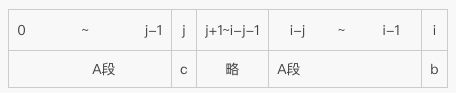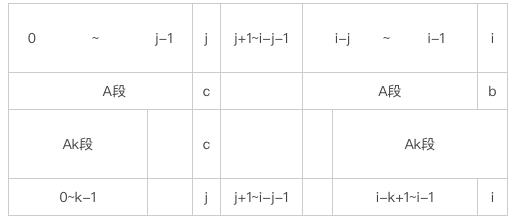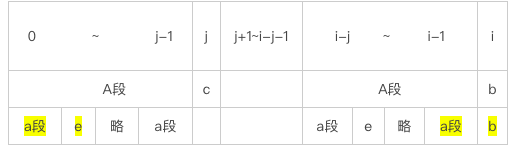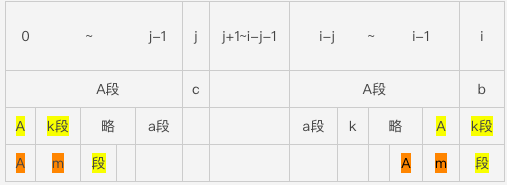# KMP算法中get_next函数分析

2017/05/16 11:45

get_next函数:

void get_next(int T[],int next[]){
int i=0;
next=-1;
int j=-1;
while (T[i]!='\0') {
if (j==-1||T[i]==T[j]) {
i++;j++; next[i]=j;
}else{
j=next[j];
}
}
}

i：i是一个位置指针，移动过程就是从左到右，依次增加，T [i]代表的就是T的第i位字符（从0开始）

next [i]：代表第0位到i-1位的最长相同前后缀的长度

j：j代表的意义比较多样，我们可以看到函数中j又用来当做位置指针（如：T[i]与T[j]进行比较），又用来当做最长相同前后缀的长度（如：用来给next[i]进行赋值），最奇怪的是还用next[j]给自身赋值（如：j=next[j]，这里其实是一个回溯的过程，j作为回溯的步长，下面会讲到）短短的几行代码，j简直了，无所不能，哪都有他，你咋不上天呢？我们接下来会着重分析一下j到底为什么这么牛X。

i：一直往后走

j：如果发现字符串第j位与第i位相同，那么j就+1，也就是往后走一位，然后新的j位与新的i位再进行比较，如果再相同就再往后走一位，依次进行，如果比较到两个字符不同，则j进行回溯，j回到某一个位置，再与i进行比较，总之我们要确保j所在的位置，能保证0~j-1位能和i-j+1~i位字符一一对应

 i 0 1 2 3 4 5 6 T a b c a b b c next -1

 i 0 1 2 3 4 5 6 T a b c a b b c next -1 0 0

 i 0 1 2 3 4 5 6 T a b c a b b c next -1 0 0 0 1

 i 0 1 2 3 4 5 6 T a b c a b b c next -1 0 0 0 1 2 0

第一次循环 初始值：i=0 j=-1;         改变后的值：  i=1，j=0，next=0

第二次循环 初始值：i=1  j=0;          改变后的值：  j=next=-1

第三次循环 初始值：i=0 j=-1；       改变后的值：  i=2，j=0，next=0

第四次循环 初始值：i=2 j=0；        改变后的值：  j=next=-1

第五次循环 初始值：i=2  j=-1；      改变后的值：  i=3，j=0，next=0

void get_next(int T[],int next[]){
int i=0;
next=-1;
int j=-1;
while (T[i]!='\0') {
if (j==-1||T[i]==T[j]) {//②这时j==-1
i++;j++; next[i]=j; //③i++,j+1=0,next[i]=0
}else{
j=-1;//①如果要是T[i]!=T[j]，那么我们就直接让j=-1
}
}
}

# 这是一定的吗？

 i 0 1 2 3 4 5 6 T a a b a a a c next -1 0 1 0 1 2 ?

i=4，j=0时，也就是考察0~3位，a a b a，最长相同前后缀是a，这时i++，j++

i=5，j=1时，考察0~4位，a a b a a，最长前后缀是a a ，i++，j++

i=6，j=2时，考察0~5位，a a b a a a，这时T != T,也就是说'b'！='a',那么T是不是应该等于0了呢？0
0 收藏

### 作者的其它热门文章2017/05/16 23:49

1 评论
0 收藏
0